# 9. Oscillators, part II: Uses, simplifications, and elaborations of negative feedback oscillators¶

Design principles

• Oscillator phase can be used as a growth timer.

• Ultrasensitive delayed repression produces oscillations in gene expression.

• Combination of delayed negative feedback with positive feedback enables independent tuning of oscillator period and amplitude.

Techniques

• Linear stability analysis of delay differential equations (DDEs)

• “Brute force” linear stability diagrams

• Numerical solution of DDEs

:

import numpy as np
import scipy.integrate
import scipy.interpolate
import scipy.optimize

import bokeh.io
import bokeh.plotting

bokeh.io.output_notebook()


The repressilator, introduced in the previous lecture, shows that delayed negative feedback, under the right conditions, can provide robust oscillations. Today, we will further explore this circuit design, showing how it can be used as a timer to record bacterial growth in the mammalian gut, simplified into a single gene oscillator, and extended with the addition of positive feedback to allow independent tuning of period and amplitude.

## Part I: Precision repressilators can record bacterial growth in the mammalian gut¶

This section is based on Riglar DT et al, “Bacterial variability in the mammalian gut captured by a single-cell synthetic oscillator,” Nature Communications, 2019.

In the previous lecture, we introduced the repressilator, a cyclic “rock-scissors-papers” feedback loop, in which three repressors cyclically repress each other. We also discussed improved versions of the repressilator developed by Potvin-Trottier et al. Some variants of the circuit they generated achieved both long periods and astonishing precision. For example, one variant had a period of 14 cell cycles! The improvements in the repressilator are summarized in an image related to this accompanying perspective.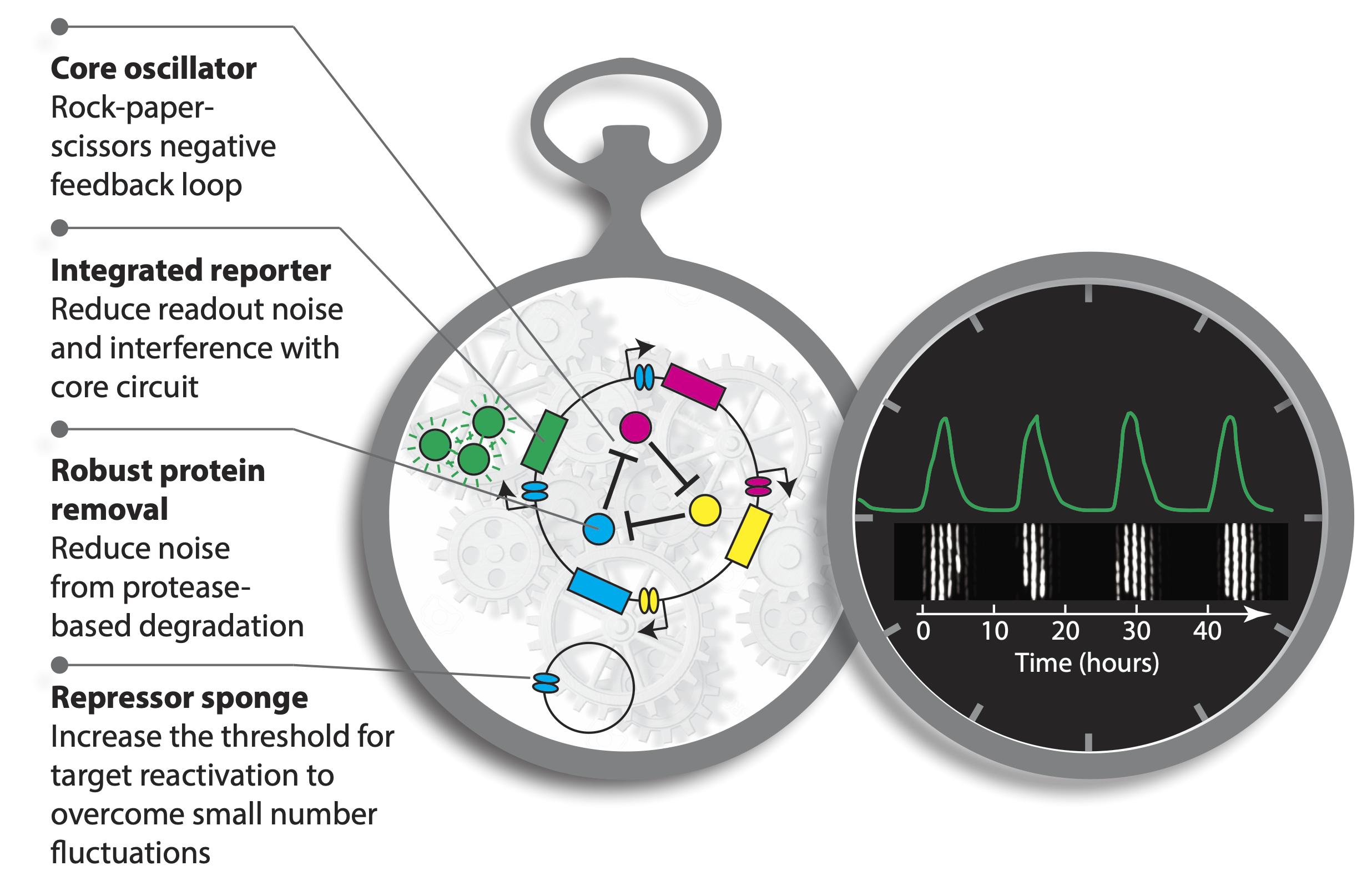The long period repressilators were enabled in part by not destabilizing the proteins, making dilution through growth the only mechanism of protein removal. Despite their long periods, this circuit was incredibly precise. The standard deviation in period length was about 14%, and the pulse shape and amplitude was incredibly regular, as you can see in the image below.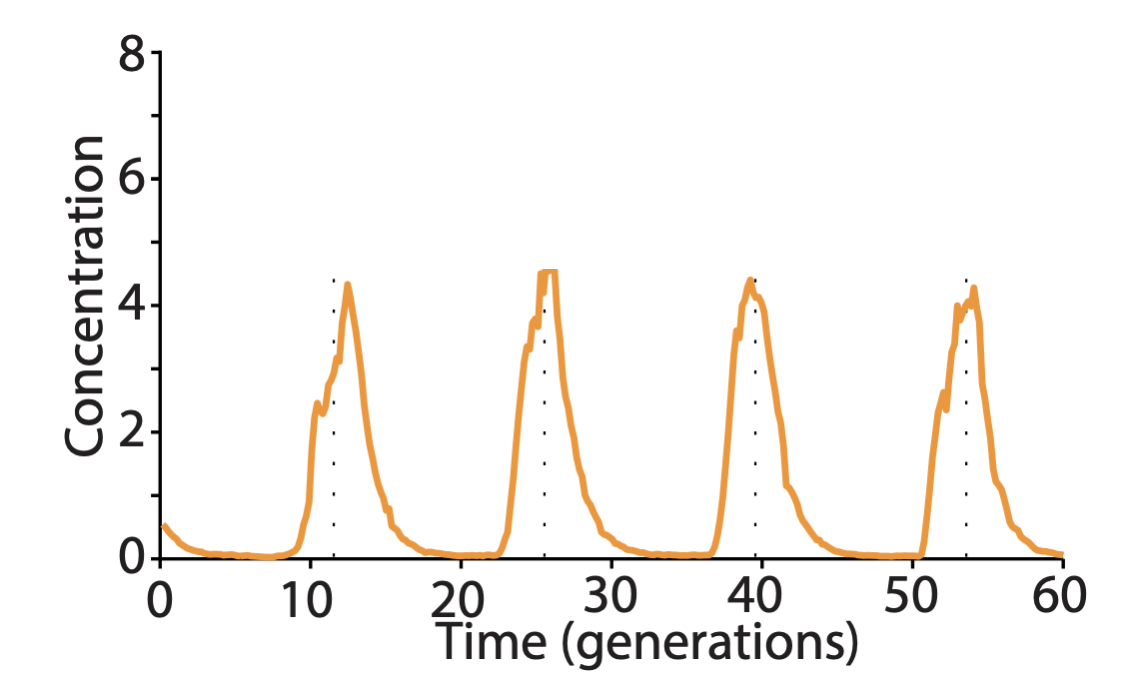In fact, this circuit is so precise that even though cells are not synchronized with one another, they stay in sync over many generations of growth. When the cells grow as a colony on a plate, most growth occurs at the leading edge of the colony. Behind that front, cells stop growing, effectively leaving a record of the state of their repressilators at each point in time, somewhat like tree rings, as shown in this image: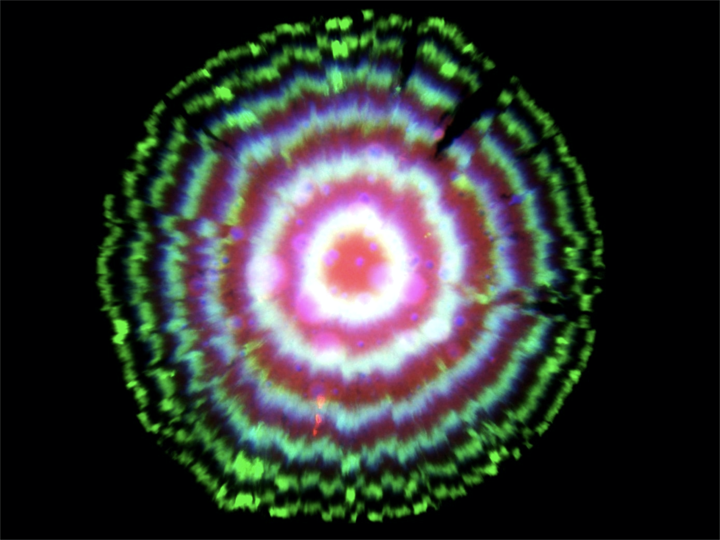One might have imagined that increasing the precision of the repressilator would require additional regulatory circuitry, but this does not seem to be the case, at least under these well-controlled conditions. As we wrote in an accompanying piece, “Evidently, precision does not necessarily demand circuit complexity and, in this case, even seems to benefit from minimalism.”. At the same time, achieving a constant period irrespective of growth conditions will likely require compensation mechanisms, much as early navigational clocks required additional systems to compensate for the effects of varying temperature on clock period.

### Ring phases enable inference of bacterial growth dynamics¶

Last year, Pam Silver and colleagues took advantage of the incredible precision offered by this circuit to interrogate bacterial growth dynamics in the mammalian gut Riglar, et al., 2019. Their approach relied on the ability to read out repressilator phase from colony ring phases. More specifically, they found that the phase of the repressilator in the initial cell that seeded the colony controls the radial phasing of the rings in the colony. Cells that are plated at the peak of reporter fluorescence produce colonies with a high intensity “dot” at the center, whereas cells plated at the trough of the oscillations have rings shifted by 180 degrees. This is shown schematically in part c of the image below. They also took advantage of the ability to modulate the phase of the repressilator directly with two small molecule drugs, anhydrotetracycline (aTc) and IPTG, which respectively inhibit TetR and LacI proteins (a, below). After using these drugs to synchronize a population of cells at one phase, they could observe a beautiful linear relationship between the repressilator phase (as determined by the phasing of colony rings) and the elapsed growth of the cells (bottom-right plot, below). Further, they verified that it was really growth and not time per se that correlated with phase (see Figure 3 of the paper).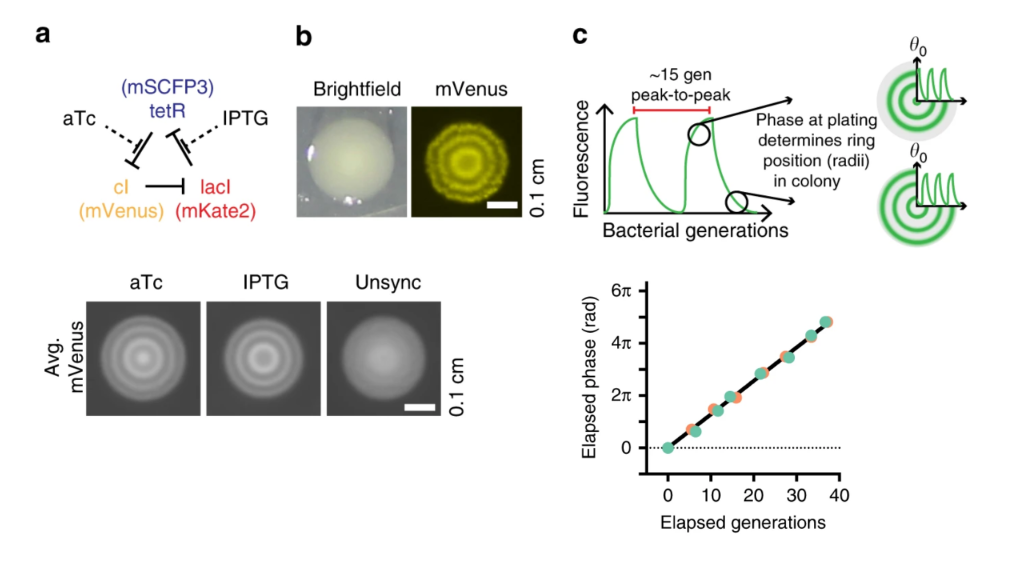Colony phasing indicates cumulative cell growth. (a) The repressilator can be perturbed by adding the drugs aTc and IPTG, which inhibit TetR and LacI, respectively. This allows one to put the system into a defined phase. (b) Colony showing the rings of fluorescent protein expression that indicate phase. (c) Schematic indicating how repressilator phase correlates with colony ring phase. (lower left) Examples of colonies generated by cells exposed to either aTc or IPTG. Note the distinct phasing. (lower right) Colony ring phase correlates with number of generations of growth.

Taken together, this means that the phase of the oscillator can be used as a measure of bacterial growth. The repressilator has become a growth readout.

They wondered whether this might allow one to recover the history of cell growth in an environment that would otherwise be inaccessible. E. coli normally spend part of their life cycle in mammalian gut, but, as you can imagine, it’s hard to observe what’s going on there directly. If we could see what was going on, it would both provide insight into how much cells typically grow within the gut, how much cell-cell variability there is in that growth, and how the amount of growth depends on conditions in the gut, including which other bacteria are present, or whether it has been recently depleted of bacteria by antibiotics. In fact, with this approach, they identified conditions, such as inflammation, that led to greater variability in growth. They also saw that growth varied between different spatial areas or niches in the gut.

This work demonstrates a few key points:

• Even a relatively simple synthetic circuit of three genes can operate with incredible precision in a living cell.

• Synthetic circuits like the repressilator can provide unique functionality both to analyze biological systems (as in this example) and, increasingly, to provide useful new functions such as therapeutics. In this case, one could imagine further exploring how engineered probiotic cells behave when introduced into animals.

• The three-repressor structure of the repressilator, and the way its dynamics progress sequentially from expression of one repressor to the next, means that one can define the phase of the oscillator. Phase is a critical aspect of many natural biological oscillators. For example, the cell cycle is controlled by an oscillator that advances a cell from one phase to the next, in a sequential cycle, with each phase performing unique activities. These phases begin with growth during G1 phase, followed by DNA replication during S phase, a second G phase (G2), and finally an M phase corresponding to mitosis.

## Part II: Less is more, or the simplest oscillator¶

Next, we continue our discussion of oscillators by considering a very simple oscillator, even simpler than the repressilator, but based on a similar property of delayed negative feedback. Specifically, we consider a single-gene circuit with negative feedback with delay.

The basic idea has been put forward multiple times, notably by Julian Lewis in 2003, describing transcriptional delay.

Delayed negative feedback is actually quite familiar to anyone who has encountered a poorly designed hotel room thermostat. The thermostat will turn on the heater in response to cold temperatures, overheating the room beyond the set point before shutting off and letting it cool well below the target temperature before turning the heater back on. These types of overshoot dynamics are usually regarded as a problem to be eliminated by more sophisticated controller designs—-the major subject of control theory.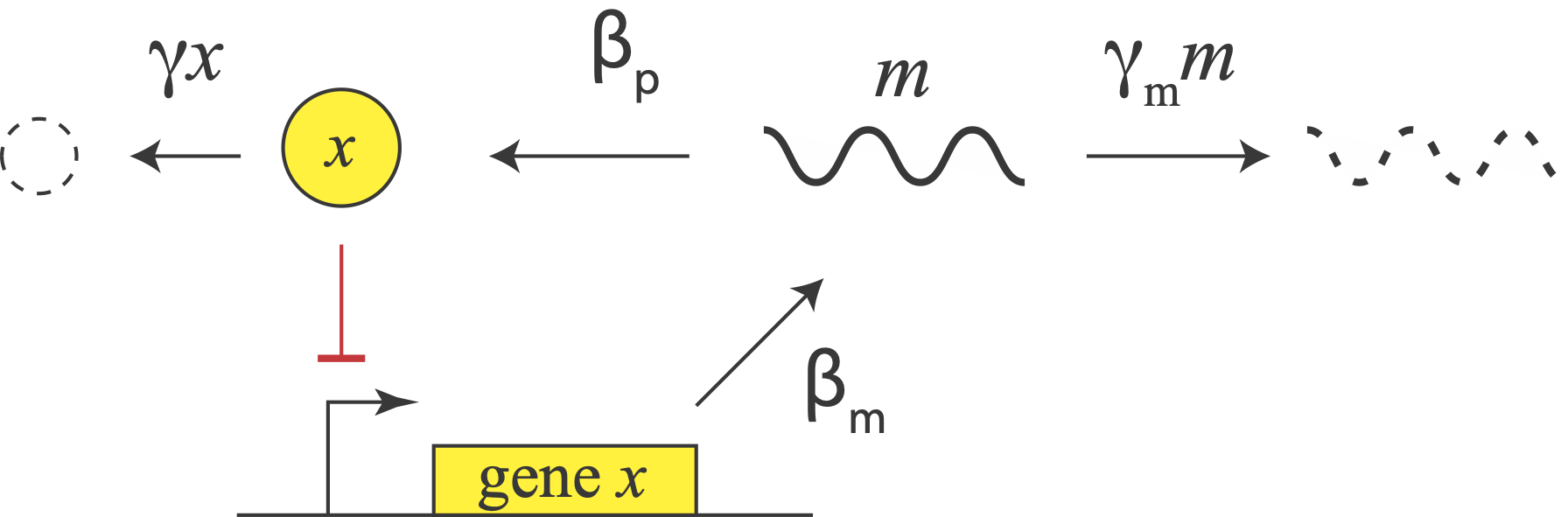Let us consider a repressor that negatively autoregulates its own production, as in the diagram. The concentration of the protein does not instantaneously reflect the expression level of its own gene. For one thing, there are the transcription and translation steps we have already encountered. But there is also a second source of delay. The appearance of a freshly translated protein does not actually occur until translation is complete. That is, there is a delay between the initiation and completion of translation for each protein. (By contrast, translation can occur co-transcriptionally in bacteria, so translation does not have to wait to start before transcription is finished.)

In E. coli, translation occurs at a rate of about 15 amino acids per second. So a sizable protein like β-galactosidase can take more than a minute to translate.

In eukaryotic cells, where gene expression has additional steps, including splicing and nuclear export, these delays can be even longer. In fact, a totally different synthetic single gene negative autoregulation oscillator circuits was implemented in eukaryotic cells using the variability in intron length (Swinburne, et al., Genes and Dev., 2008), rather than translational delay per se, to delay protein production.

## An example of delay in a biological circuit¶

To investigate how molecular details can give rise to delay (which in turn can give oscillations), we consider a multistep process of the production and action of a repressor. The delay in transcriptional regulation in real cells comes from processes such as translation, trans-nuclear transport, etc. We will consider a simple version of this, shown below, in which $$r$$ is some intermediate state en route to functioning protein.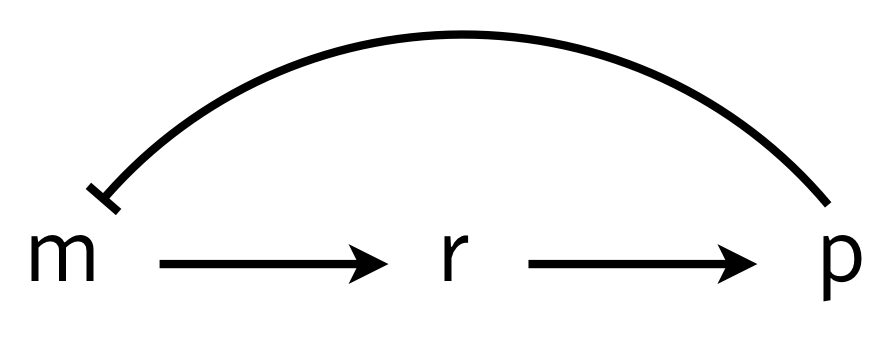We can write the dynamics as

\begin{align} \frac{\mathrm{d}m}{\mathrm{d}t} &= \frac{\beta_{m}}{1 + (p/k)^n} - \gamma_m m,\\[1em] \frac{\mathrm{d}r}{\mathrm{d}t} &= \beta_{r} m - \gamma_r r,\\[1em] \frac{\mathrm{d}p}{\mathrm{d}t} &= \beta_{p} r - \gamma_{p} p, \end{align}

where for simplicity we have assumed mass action type kinetics for all processes other than repression. These equations can be nondimensionalized by renaming parameters and variables as $$r \leftarrow \gamma_m t$$, $$p \leftarrow p/k$$, $$m \leftarrow \beta_{r}\beta_{p} m/\gamma_m^2 k$$, $$r \leftarrow \beta_{p} r/\gamma_m k$$, $$\beta \leftarrow \beta_{m} \beta_{r} \beta_{p}/\gamma_m^2 k$$ and $$\gamma \leftarrow \gamma_{p}/\gamma_m$$ to give

\begin{align} \frac{\mathrm{d}m}{\mathrm{d}t} &= \frac{\beta}{1 + p^n} - m,\\ \frac{\mathrm{d}r}{\mathrm{d}t} &= m - r,\\ \frac{\mathrm{d}p}{\mathrm{d}t} &= r - \gamma p. \end{align}

There is a single fixed point $$(m_0, r_0, p_0)$$ for this system,

\begin{align} m_0 &= r_0 = \gamma p_0,\\ \frac{\beta}{\gamma} &= p_0(1+p_0^n). \end{align}

We can perform linear stability analysis about this fixed point, just as we did in for the repressilator.

\begin{align} \frac{\mathrm{d}}{\mathrm{d}t}\begin{pmatrix} \delta m \\ \delta r \\ \delta p \end{pmatrix} = \mathsf{A}\cdot\begin{pmatrix} \delta m \\ \delta r \\ \delta p \end{pmatrix}, \end{align}

with

\begin{align} \mathsf{A} = \begin{pmatrix} -1 & 0 & -a \\ 1 & -1 & 0 \\ 0 & 1 & -\gamma \end{pmatrix}, \end{align}

where

\begin{align} a = \frac{\beta n p_0^{n-1}}{(1+p_0^n)^2} = \frac{\gamma n p_0^n}{1+p_0^n}. \end{align}

To find the eigenvalues, we write the characteristic polynomial as

\begin{align} -(1+\lambda)^2(\gamma+\lambda) - a = -\lambda^3 - (2+\gamma)\lambda^2 - (1+2\gamma)\lambda -\gamma - a = 0. \end{align}

This polynomial has no positive real roots and either one or three negative real roots according to the Descartes Sign Rule. We are interested in the case where we have one negative real root and two complex conjugate roots. If the real part of these complex conjugate roots is positive, we have an oscillatory instability.

We can compute the eigenvalues of the linear stability matrix for various values of $$\beta$$ and $$\gamma$$ for fixed Hill coefficient $$n$$. We will take a “brute force” approach and grid up $$\beta$$-$$\gamma$$ space and compute the eigenvalues for each set of parameter values and determine if that point is stable or not. We can then display the linear stability diagram as an image, where a pixel is light colored in a stable region and dark colored in an unstable region.

:

def fixed_point(beta, gamma, n):
"""Computes fixed point the m⟶r⟶p autorepression system.
Uses np.roots because it has better convergence properties than
scipy.optimize.fixed_point for integer n.
"""
coeffs = np.zeros(n + 1)
coeffs = 1
coeffs[-2] = 1
coeffs[-1] = -beta / gamma
p_0 = np.roots(coeffs)

return p_0[(p_0.imag == 0) & (p_0 > 0)].real

def lin_stab(beta, gamma, n):
"""Returns True if delay system is linearly stable and False
otherwise.
"""
p_0 = fixed_point(beta, gamma, n)

# Linear stability matrix
a = gamma * n * p_0 ** n / (1 + p_0 ** n)
L = np.array([[-1, 0, -a], [1, -1, 0], [0, 1, -gamma]])

# Compute eigenvalues
lam = np.linalg.eigvals(L)

return (lam.real < 0).all()

# Ultrasensitivity
n = 10

#
beta_vals = np.logspace(0, 4, 400)
gamma_vals = np.logspace(-1, 2, 400)

# Compute the linear stability diagram
stab = np.empty((len(beta_vals), len(gamma_vals)))
for i, beta in enumerate(beta_vals):
for j, gamma in enumerate(gamma_vals):
stab[i, j] = lin_stab(beta, gamma, n)

# Plot the result (shaded region has oscillatory instability)
p = bokeh.plotting.figure(
plot_height=300,
plot_width=350,
x_axis_label="γ",
y_axis_label="β",
x_axis_type="log",
y_axis_type="log",
x_range=bokeh.models.Range1d(0.1, 100),
y_range=bokeh.models.Range1d(1, 10000),
)
p.image([stab], x=0.1, y=1, dw=100, dh=10000, palette="Greys3", alpha=0.5)
p.text(x=0.45, y=100, text=["unstable"])
p.text(x=10, y=2, text=["stable"])

bokeh.io.show(p)